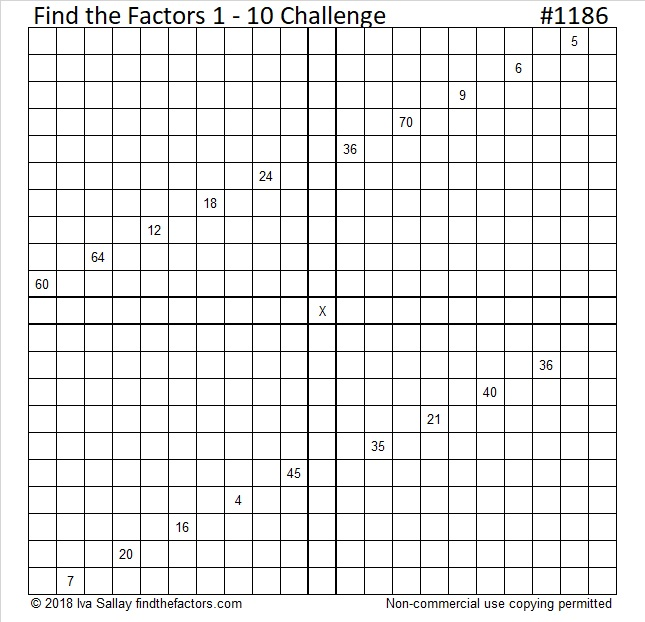# 1186 Challenge Puzzle

It shouldn’t be too hard to make your first move in this puzzle. After that, I don’t make any guarantees. You just need to write each number from 1 to 10 in each of the four boldly outlined areas so that the given clues are the products of the factors you wrote. Use logic to find all the factors and have fun doing it!Print the puzzles or type the solution in this excel file: 10-factors-1174-1186

What have I found out about the number 1186?

• 1186 is a composite number.
• Prime factorization: 1186 = 2 × 593
• The exponents in the prime factorization are 1 and 1. Adding one to each and multiplying we get (1 + 1)(1 + 1) = 2 × 2 = 4. Therefore 1186 has exactly 4 factors.
• Factors of 1186: 1, 2, 593, 1186
• Factor pairs: 1186 = 1 × 1186 or 2 × 593
• 1186 has no square factors that allow its square root to be simplified. √1186 ≈ 34.4383531² + 15² = 1186

1186 is the hypotenuse of a Pythagorean triple:
736-930-1186 calculated from 31² – 15², 2(31)(15), 31² + 15²

1186 is palindrome 989 in BASE 11

This site uses Akismet to reduce spam. Learn how your comment data is processed.